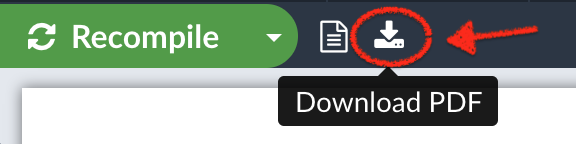## 什么是 LaTeX ？

LaTeX （发音为 LAY-tek or LAH-tek） 是个用于创建专业外观文档的工具。

## 为什么学习 LaTeX ？

LaTeX 在世界范围内用于科学文档，书籍以及许多其他形式的出版。

## 来写你的第一篇 LaTeX 吧

\documentclass{article}

\begin{document}
First document. This is a simple example, with no
extra parameters or packages included.
\end{document}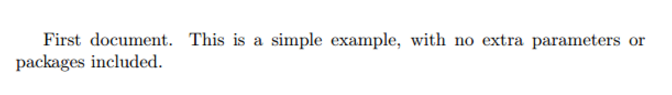Open an example in Overleaf

（你也可以通过点击 Recompile 按钮旁的小尖头，并设置 Auto Compile 为开，来将项目设置为在编辑文件时自动重新编译。）

## 一篇文档的序文

preamble 中，你定义要编写的文档的类型，编写所用的语言，想要使用的包（稍后会详细介绍）和其他几个元素。

\documentclass[12pt, letterpaper]{article}
\usepackage[utf8]{inputenc}

\documentclass[12pt, letterpaper]{article}

\usepackage[utf8]{inputenc}

## 添加标题、作者和日期

\title{First document}

\author{Hubert Farnsworth}

\thanks{funded by the Overleaf team}

\date{February 2014}

\documentclass[12pt, letterpaper, twoside]{article}
\usepackage[utf8]{inputenc}

\title{First document}
\author{Hubert Farnsworth \thanks{funded by the Overleaf team}}
\date{February 2017}

\begin{document}

\maketitle

We have now added a title, author and date to our first \LaTeX{} document!

\end{document}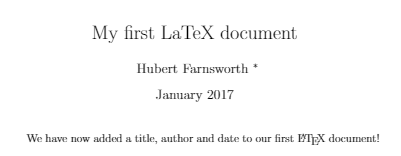Open an example in Overleaf

## 添加注释

\begin{document}

\maketitle

We have now added a title, author and date to our first \LaTeX{} document!

% This line here is a comment. It will not be printed in the document.

\end{document}Open an example in Overleaf

## 粗体，斜体和下划线

• 粗体：LaTeX 中的粗体文本使用 \textbf{...} 命令编写。
• _斜体_：LaTeX 中的斜体文本使用 \textit{...} 命令编写。
• 下划线：LaTeX 中带下划线的文本使用 \underline{...} 命令编写。

Some of the \textbf{greatest}
discoveries in \underline{science}
were made by \textbf{\textit{accident}}.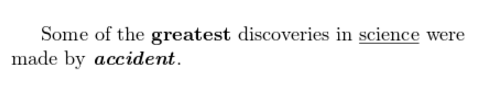Some of the greatest \emph{discoveries}
in science

\textit{Some of the greatest \emph{discoveries}
in science

\textbf{Some of the greatest \emph{discoveries}
in science
were made by accident.}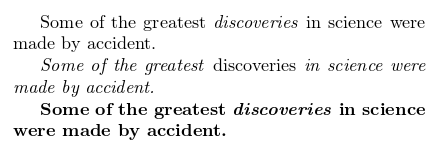Open an example in Overleaf

## 添加图像

\documentclass{article}
\usepackage{graphicx}
\graphicspath{ {images/} }

\begin{document}
The universe is immense and it seems to be homogeneous,
in a large scale, everywhere we look at.

\includegraphics{universe}

There's a picture of a galaxy above
\end{document}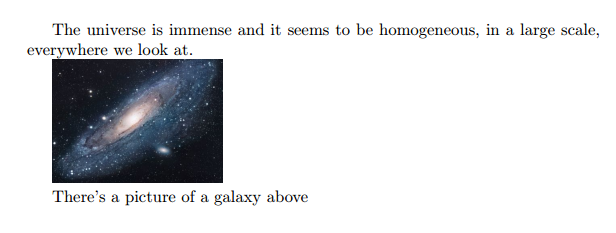Open an example in Overleaf

LaTeX 不能单独管理图像，因此您需要使用一个包。包可用于更改 LaTeX 文档的默认外观，或允许更多功能。

\includegraphics{universe} 是将图像实际包含在文档中的命令。

## 标题，标签和参考

\begin{figure}[h]
\centering
\includegraphics[width=0.25\textwidth]{mesh}
\caption{a nice plot}
\label{fig:mesh1}
\end{figure}

As you can see in the figure \ref{fig:mesh1}, the
function grows near 0. Also, in the page \pageref{fig:mesh1}
is the same example.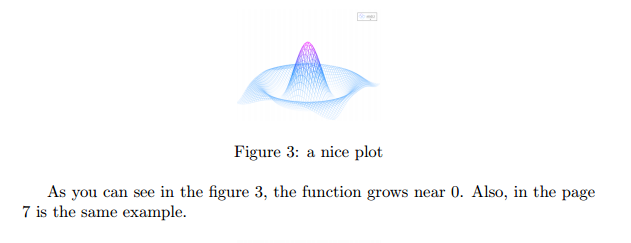Open an example in Overleaf

• \caption{a nice plot}：您可能会期望此命令为图形设置标题。如果你创建了一个图形列表，那么标题将会被用在这里。您可以将其放在图的上方或下方。
• \label{fig:mesh1}：如果您需要在文档中引用图像，请使用此命令设置标签。标签将为图像编号，并与下一个命令结合使用，以供您参考。
• \ref{fig:mesh1}：该代码将替换为与参考图相对应的数字。

## 在 LaTeX 中创建列表

### 无序列表

\begin{itemize}
\item The individual entries are indicated with a black dot, a so-called bullet.
\item The text in the entries may be of any length.
\end{itemize}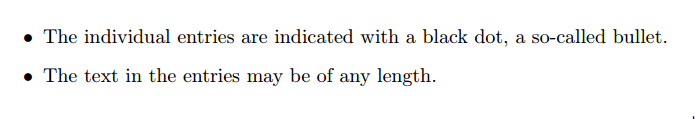Open an example in Overleaf

### 有序列表

\begin{enumerate}
\item This is the first entry in our list
\item The list numbers increase with each entry we add
\end{enumerate}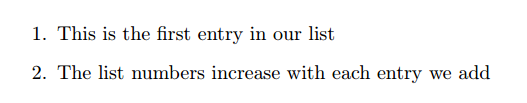Open an example in Overleaf

## 为 LaTeX 添加数学符号

LaTeX 的主要优点之一是易于编写数学表达式。
LaTeX 允许两种用于数学表达式的书写模式：inline（内联）模式和 display（显示）模式。

In physics, the mass-energy equivalence is stated
by the equation $E=mc^2$, discovered in 1905 by Albert Einstein.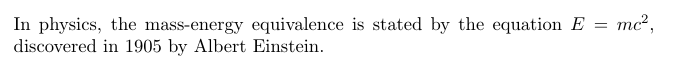displayed 模式有两种版本：已编号和未编号。

The mass-energy equivalence is described by the famous equation

$E=mc^2$

discovered in 1905 by Albert Einstein.
In natural units ($c = 1$), the formula expresses the identity

\begin{equation}
E=m
\end{equation}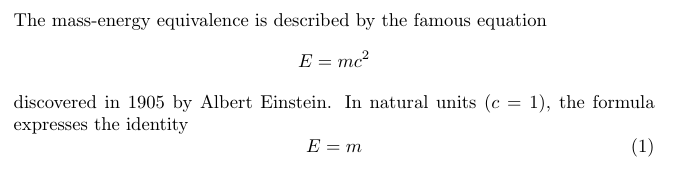Open an example in Overleaf

Subscripts in math mode are written as $a_b$ and superscripts are written as $a^b$. These can be combined an nested to write expressions such as

$T^{i_1 i_2 \dots i_p}_{j_1 j_2 \dots j_q} = T(x^{i_1},\dots,x^{i_p},e_{j_1},\dots,e_{j_q})$

We write integrals using $\int$ and fractions using $\frac{a}{b}$. Limits are placed on integrals using superscripts and subscripts:

$\int_0^1 \frac{1}{e^x} = \frac{e-1}{e}$

Lower case Greek letters are written as $\omega$ $\delta$ etc. while upper case Greek letters are written as $\Omega$ $\Delta$.

Mathematical operators are prefixed with a backslash as $\sin(\beta)$, $\cos(\alpha)$, $\log(x)$ etc.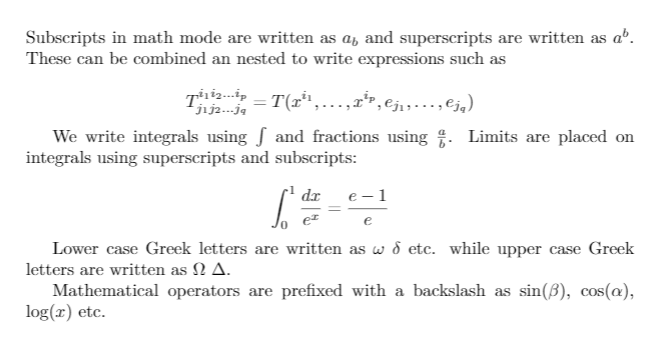Open an example in Overleaf

## 基本格式

### 摘要

\begin{document}

\begin{abstract}
This is a simple paragraph at the beginning of the
document. A brief introduction about the main subject.
\end{abstract}
\end{document}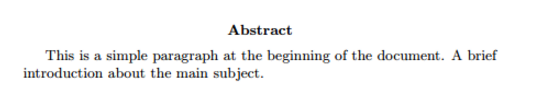Open an example in Overleaf

### 段落和换行符

\begin{document}

\begin{abstract}
This is a simple paragraph at the beginning of the
document. A brief introduction about the main subject.
\end{abstract}

Now that we have written our abstract, we can begin writing our first paragraph.

This line will start a second Paragraph.
\end{document}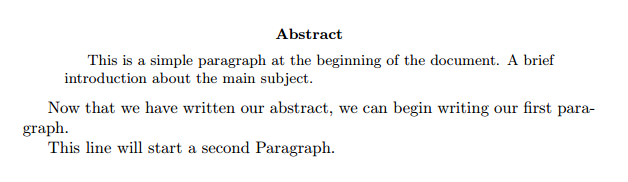Open an example in Overleaf

### 章节

\chapter{First Chapter}

\section{Introduction}

This is the first section.

Lorem  ipsum  dolor  sit  amet,  consectetuer  adipiscing
elit.   Etiam  lobortisfacilisis sem.  Nullam nec mi et
neque pharetra sollicitudin.  Praesent imperdietmi nec ante.
Donec ullamcorper, felis non sodales...

\section{Second Section}

Lorem ipsum dolor sit amet, consectetuer adipiscing elit.
Etiam lobortis facilisissem.  Nullam nec mi et neque pharetra
sollicitudin.  Praesent imperdiet mi necante...

\subsection{First Subsection}
Praesent imperdietmi nec ante. Donec ullamcorper, felis non sodales...

\section*{Unnumbered Section}
Lorem ipsum dolor sit amet, consectetuer adipiscing elit.
Etiam lobortis facilisissem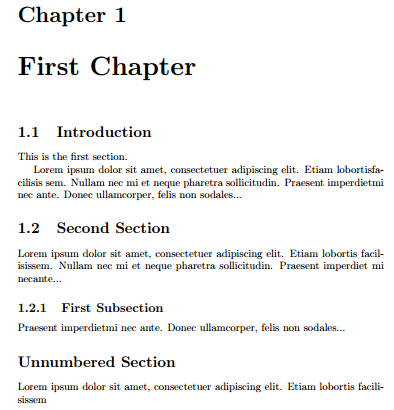Open an example in Overleaf

-1 \part{part}
0 \chapter{chapter}
1 \section{section}
2 \subsection{subsection}
3 \subsubsection{subsubsection}
4 \paragraph{paragraph}
5 \subparagraph{subparagraph}

## 创建表格

### 在 LaTeX 中创建一个简单的表

\begin{center}
\begin{tabular}{ c c c }
cell1 & cell2 & cell3 \\
cell4 & cell5 & cell6 \\
cell7 & cell8 & cell9
\end{tabular}
\end{center}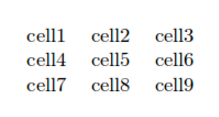tabular（表格）环境是 LaTeX 创建表格的默认方法。您必须为此环境指定一个参数，在这种情况下为 {c c c}

Open an example in Overleaf

### 添加边框

tabular 环境更加灵活，您可以在每列之间放置分隔线。

\begin{center}
\begin{tabular}{ |c|c|c| }
\hline
cell1 & cell2 & cell3 \\
cell4 & cell5 & cell6 \\
cell7 & cell8 & cell9 \\
\hline
\end{tabular}
\end{center}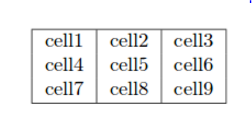• { |c|c|c| }：这声明了被垂直线分割的三列，并将被应用在表格中。| 符号指定这些列应由一条垂直线分隔。
• \hline：这将插入一条水平线。在这里，我们在表格的顶部和底部包括了水平线。你使用 \hline 的次数没有限制。

\begin{center}
\begin{tabular}{||c c c c||}
\hline
Col1 & Col2 & Col2 & Col3 \\ [0.5ex]
\hline\hline
1 & 6 & 87837 & 787 \\
\hline
2 & 7 & 78 & 5415 \\
\hline
3 & 545 & 778 & 7507 \\
\hline
4 & 545 & 18744 & 7560 \\
\hline
5 & 88 & 788 & 6344 \\ [1ex]
\hline
\end{tabular}
\end{center}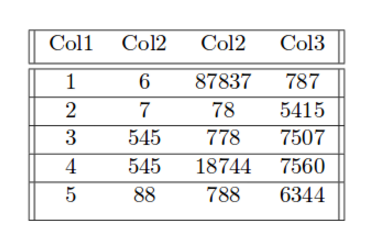Open an example in Overleaf

### 表格说明（标题），标签和参考

Table \ref{table:data} is an example of referenced \LaTeX{} elements.

\begin{table}[h!]
\centering
\begin{tabular}{||c c c c||}
\hline
Col1 & Col2 & Col2 & Col3 \\ [0.5ex]
\hline\hline
1 & 6 & 87837 & 787 \\
2 & 7 & 78 & 5415 \\
3 & 545 & 778 & 7507 \\
4 & 545 & 18744 & 7560 \\
5 & 88 & 788 & 6344 \\ [1ex]
\hline
\end{tabular}
\caption{Table to test captions and labels}
\label{table:data}
\end{table}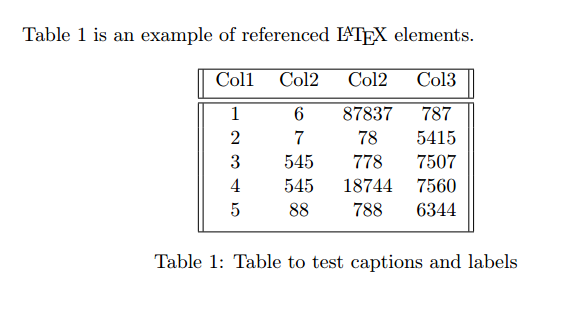Open an example in Overleaf

### 添加目录

\documentclass{article}
\usepackage[utf8]{inputenc}

\title{Sections and Chapters}
\author{Gubert Farnsworth}
\date{ }

\begin{document}

\maketitle

\tableofcontents

\section{Introduction}

This is the first section.

Lorem  ipsum  dolor  sit  amet,  consectetuer  adipiscing
elit.   Etiam  lobortisfacilisis sem.  Nullam nec mi et
neque pharetra sollicitudin.  Praesent imperdietmi nec ante.
Donec ullamcorper, felis non sodales...

\section*{Unnumbered Section}

Lorem ipsum dolor sit amet, consectetuer adipiscing elit.
Etiam lobortis facilisissem.  Nullam nec mi et neque pharetra
sollicitudin.  Praesent imperdiet mi necante...

\section{Second Section}

Lorem ipsum dolor sit amet, consectetuer adipiscing elit.
Etiam lobortis facilisissem.  Nullam nec mi et neque pharetra
sollicitudin.  Praesent imperdiet mi necante...

\end{document}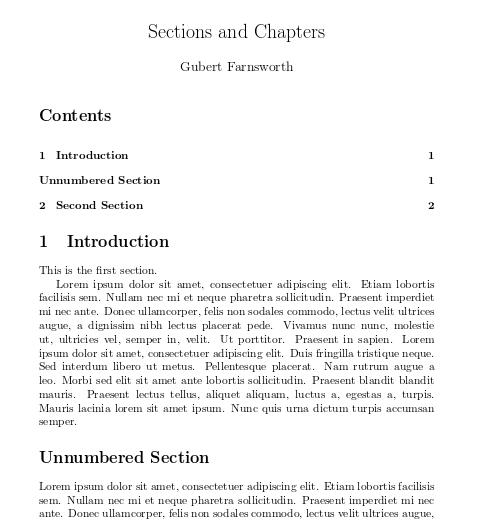Open an example in Overleaf

## 下载你完成的文件